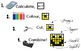# Colouring by Linear Systems, Batman vs Superman (Two 12 Sheet Mosaics)Subject
Resource Type
File Type

Zip

(2 MB|53 pages)
Standards
• Product Description
• Standards

Here is the point of intersection between Math and Justice! Linear systems meet superheroes in a collaboratively engaging math class colouring battle! Two 12-sheet mosaics are included (Superman and Batman), so you can split up your students and have a substitution-and-elimination-filled showdown! May the best coloured hero win! Each student's worksheet represents a small section of the larger hero mosaic.

Each mosaic consists of 12 worksheets (each one unique!) containing 12 linear system problems. The problems are designed to encourage students to choose the most appropriate strategy (substitution, elimination, or graphing). Students solve for the point of intersection of each system, then use the specified coordinate to colour their worksheets based on the colour key. Students' sheets combine to create the hero mosaics.

Get this product as part of a MASSIVE linear systems bundle!

The collaborative motivation is huge!

The answers are given in a randomized list at the bottom of each sheet, allowing students to monitor their own work. But complete answer keys are provided for you, as well as a Teaching Tips page for smooth implementation!

This product comes in both .pdf and .docx format. All files are included in the zip folder.

CHECK OUT THE PRODUCT PREVIEW to see the range of problem types and know exactly what these worksheets involve!

The Superman vs Batman battle is also available in the following versions:

Fractions - +,-, ×, and ÷ positive mixed fractions

Sine and Cosine Laws - solve for sides and angles

Trig Ratios (SohCahToa) - solve for sides and angles

Pythagorean Theorem - word problems and life applications

It's simple!

2. Colour the squares.

4. Combine with the class!

Both a visual answer key, showing the completed mosaic with labelled coordinates (to help you assemble the picture from the combined worksheets), and a numeric answer key for each individual worksheet are provided for both hero mosaics.

Leave the hero pictures a secret, or let them know their team in advance for motivation… it’s your call.

Encourage students to check their answers by finding them in the randomized list on their worksheet before they colour each square. This will increase the accuracy of the final picture!

All my “Colouring by…” worksheets use standard pencil-crayon colours found in the Crayola 24 pack. For best results, use the exact colour name match (and encourage quality colouring!). Perhaps a class set of pencil crayons would be a fun math department investment!

Feedback, suggestions, and frontline stories are always welcomed!

COMMON CORE MATH STANDARDS:

◾ HSA.REI.C.6

Solve systems of linear equations exactly and approximately (e.g., with graphs), focusing on pairs of linear equations in two variables

◾ 8.EE.C.8

Analyze and solve pairs of simultaneous linear equations.

◾ 8.EE.C.8.a

Understand that solutions to a system of two linear equations in two variables correspond to points of intersection of their graphs, because points of intersection satisfy both equations simultaneously.

◾ 8.EE.C.8.b

Solve systems of two linear equations in two variables algebraically, and estimate solutions by graphing the equations.

◾ MPM2D, Analytic Geometry Strand

Overall Expectation AG1:

Model and solve problems involving the intersection of two straight lines

Specific Expectation:

AG1.1 Solve systems of two linear equations involving two variables, using the algebraic method of substitution or elimination

◾MFM2P Modeling Linear Relations Strand

Overall Expectation MLR3

Solve systems of two linear equations, and solve related problems that arise from realistic situations.

Specific Expectation

MLR3.1 determine graphically the point of intersection of two linear relations

MLR3.2 solve systems of two linear equations involving two variables with integral coefficients, using the algebraic method

of substitution or elimination

Thanks for checking this out!

~CalfordMath

If you like this product, be sure the check out the whole Collaborative Math Mosaic directory, sorted by topic!

Solve systems of linear equations exactly and approximately (e.g., with graphs), focusing on pairs of linear equations in two variables.
Solve systems of two linear equations in two variables algebraically, and estimate solutions by graphing the equations. Solve simple cases by inspection. For example, 3𝘹 + 2𝘺 = 5 and 3𝘹 + 2𝘺 = 6 have no solution because 3𝘹 + 2𝘺 cannot simultaneously be 5 and 6.
Understand that solutions to a system of two linear equations in two variables correspond to points of intersection of their graphs, because points of intersection satisfy both equations simultaneously.
Analyze and solve pairs of simultaneous linear equations.
Total Pages
53 pages
Included
Teaching Duration
2 days
Report this Resource to TpT
Reported resources will be reviewed by our team. Report this resource to let us know if this resource violates TpT’s content guidelines.